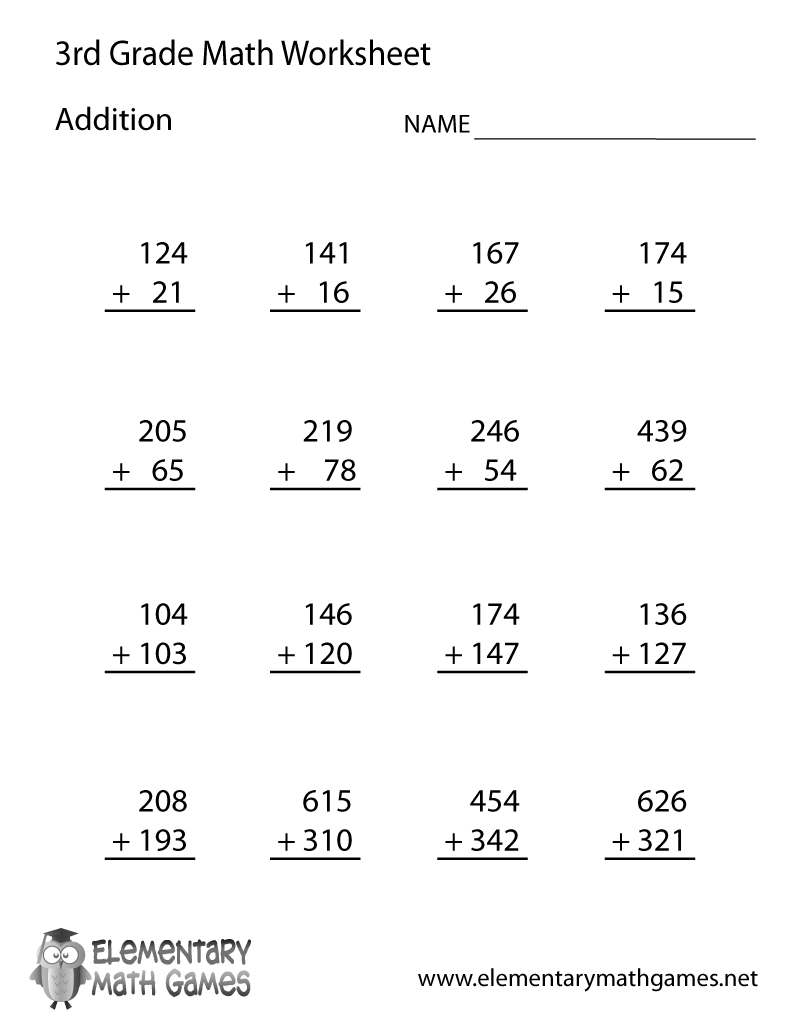This third grade math worksheet will help children learn how to add two and three digit numbers. There are 16 addition problems for third graders to solve.

Here are the two versions of this free third grade math worksheet: